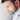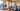Navigate back to the homepage

# L1 & L2 model regularizations techniquesBipin Paul Bedi
October 12th, 2018 · 2 min readThe most difficult and time consuming activity in any machine learning project is modelling the domain. The objective of training the model is to reduce the cost function, which can have direct dependency on feature selection and their representation. The results you achieve is a function of the model features and the weights being selected. Even your framing of the problem and measures you’re using to estimate accuracy play a part. Your results are dependent on many inter-dependent properties

Feature engineering is the process of transforming raw data into features that better represent the underlying problem to the predictive models, resulting in improved model accuracy on unseen data.

You can see the dependencies in this definition:

• The performance measures you’ve chosen (RMSE? AUC?)
• The framing of the problem (classification? regression?)
• The predictive models you’re using (SVM?)
• The raw data you have selected and prepared (samples? formatting? cleaning?)

In order to create less complex model when you have a large number of features in your dataset, some of the Regularization techniques are used to address over-fitting. In general, due to the addition of regularization term, the values of weight matrices decrease because it assumes that a neural network with smaller weight matrices leads to simpler models.

`Cost function = Loss + Regularization term`

L1 and L2 are the most common types of regularization. The key difference between these two is the penalty term

L1 regularization
A regression model that uses L1 regularization technique is called Lasso Regression

`Cost function = Loss + Λ/2m * σ|weight|`

Here, lambda is the regularization parameter. It is the hyper-parameter whose value is optimized for better results. if lambda is zero then you can imagine we get back original loss. However, if lambda is very large then it will add too much weight and it will lead to under-fitting.
In L1 regularization, we penalize the absolute value of the weights. The weights may be reduced to zero here. Hence, it is very useful when we are trying to compress our model. Otherwise, we usually prefer L2 over it.

L2 regularization
A regression model model which uses L2 is called Ridge Regression.

`Cost function = Loss + Λ/2m * σ|weight|^2`

It adds “squared magnitude” of coefficient as penalty term to the loss function. L2 regularization is also known as weight decay as it forces the weights to decay towards zero (but not exactly zero).

The key difference between these techniques is that in L1 the less important feature’s coefficient are reduced to zero thus, removing some feature altogether. So, this works well for feature selection in case we have a huge number of features.

#regularization #data-modelling #feature-modelling #machine-learning

### More articles from Bipin### Developers guide to designing REST endpoints

Coding blueprint for pragmatic rest api developers

October 10th, 2018 · 4 min read### Talks & Alternate Publishing

there's zero correlation between being the best talker and having the best ideas

January 1st, 2050 · 1 min read